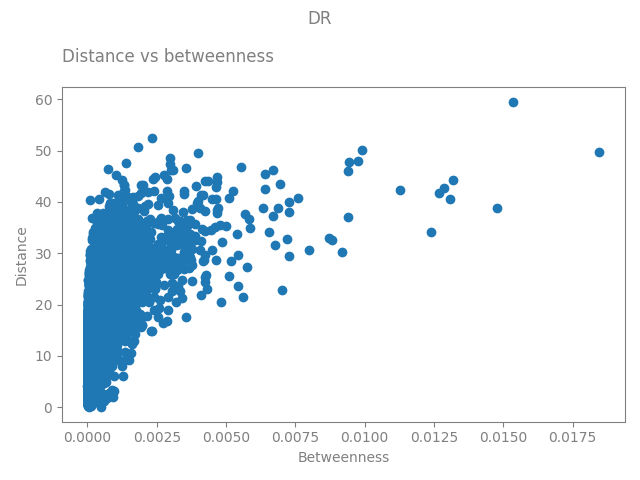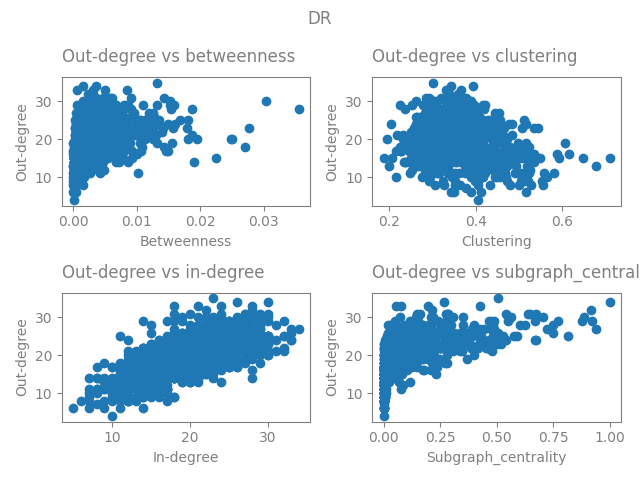# Plot various graph properties#

```import nngt
import nngt.plot as nplt
from nngt.geometry import Shape

import matplotlib.pyplot as plt

plt.rcParams.update({
'axes.edgecolor': 'grey', 'xtick.color': 'grey', 'ytick.color': 'grey',
"figure.facecolor": (0, 0, 0, 0), "axes.facecolor": (0, 0, 0, 0),
"axes.labelcolor": "grey", "text.color": "grey"
})

nngt.seed(0)
```

Let’s start by making a random exponential graph

```shape = Shape.disk(100)

g = nngt.generation.distance_rule(5, shape=shape, nodes=1000, avg_deg=20)
```

Let’s plot the distances

```nplt.edge_attributes_distribution(g, "distance", show=True)
```We then compute the betweenness and see how it correlates with the distance

```nbetw, ebetw = nngt.analysis.betweenness(g)

g.new_edge_attribute("betweenness", "float", values=ebetw)

nplt.correlation_to_attribute(g, "distance", "betweenness",
attribute_type="edge", show=True)
```Let’s check the correlations between various node properties and their degree

```g.new_node_attribute("betweenness", "float", values=nbetw)

attr = ["betweenness", "clustering", "in-degree", "subgraph_centrality"]

nplt.correlation_to_attribute(g, "out-degree", attr, show=True)
```Total running time of the script: (0 minutes 42.958 seconds)

Gallery generated by Sphinx-Gallery